## 8.1 Pressure

### Learning Objectives

By the end of this section, you will be able to:

• Define the property of pressure
• Define and convert among the units of pressure measurements

Gases were among the first substances studied using the modern scientific method, which was developed in the 1600s. It did not take long to recognize that gases all shared certain physical behaviours, suggesting that gases could be described by one all-encompassing theory. The kinetic molecular theory of gases is a model that helps us understand the physical properties of gases at the molecular level. It is based on the following concepts:

1. Gases consist of particles (molecules or atoms) that are in constant random motion.
2. Gas particles are constantly colliding with each other and the walls of their container. These collisions are elastic; that is, there is no net loss of energy from the collisions.
3. Gas particles are small and the total volume occupied by gas molecules is negligible relative to the total volume of their container.
4. There are no interactive forces (i.e., attraction or repulsion) between the particles of a gas.
5. The average kinetic energy of gas particles is proportional to the absolute temperature of the gas, and all gases at the same temperature have the same average kinetic energy.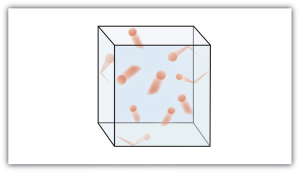Figure 1. The Kinetic Molecular Theory of Gases” shows a representation of how we mentally picture the gas phase

The kinetic molecular theory of gases describes this state of matter as composed of tiny particles in constant motion with a lot of distance between the particles. Because most of the volume occupied by a gas is empty space, a gas has a low density and can expand or contract under the appropriate influence. The fact that gas particles are in constant motion means that two or more gases will always mix as the particles from the individual gases move and collide with each other. The number of collisions the gas particles make with the walls of their container and the force with which they collide determine the magnitude of the gas pressure.

The earth’s atmosphere exerts a pressure, as does any other gas. Although we do not normally notice atmospheric pressure, we are sensitive to pressure changes—for example, when your ears “pop” during take-off and landing while flying, or when you dive underwater. Gas pressure is caused by the force exerted by gas molecules colliding with the surfaces of objects (Figure 2). Although the force of each collision is very small, any surface of appreciable area experiences a large number of collisions in a short time, which can result in a high pressure. In fact, normal air pressure is strong enough to crush a metal container when not balanced by equal pressure from inside the container.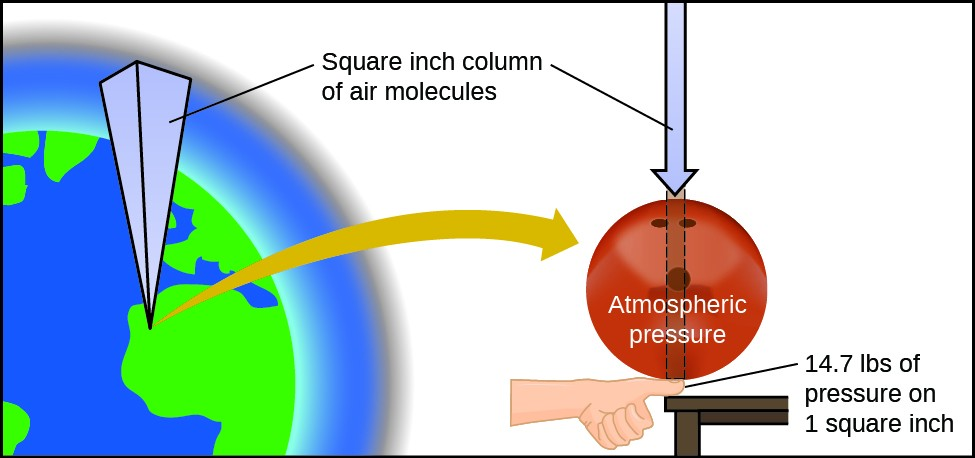Figure 2. The atmosphere above us exerts a large pressure on objects at the surface of the earth, roughly equal to the weight of a bowling ball pressing on an area the size of a human thumbnail.

A dramatic illustration of atmospheric pressure is provided in this brief video, which shows a railway tanker car imploding when its internal pressure is decreased.

A smaller scale demonstration of this phenomenon is briefly explained in the following video:

Atmospheric pressure is caused by the weight of the column of air molecules in the atmosphere above an object, such as the tanker car. At sea level, this pressure is roughly the same as that exerted by a full-grown African elephant standing on a doormat, or a typical bowling ball resting on your thumbnail. These may seem like huge amounts, and they are, but life on earth has evolved under such atmospheric pressure. If you actually perch a bowling ball on your thumbnail, the pressure experienced is twice the usual pressure (the atmospheric pressure plus the pressure of the bowling ball), and the sensation is unpleasant.

In general, pressure is defined as the force exerted on a given area: $P=\frac{F}{A}.$ Note that pressure is directly proportional to force and inversely proportional to area. Thus, pressure can be increased either by increasing the amount of force or by decreasing the area over which it is applied; pressure can be decreased by decreasing the force or increasing the area.

Let’s apply this concept to determine which would be more likely to fall through thin ice in Figure 3—the elephant or the figure skater? A large African elephant can weigh 7 tons, supported on four feet, each with a diameter of about 1.5 ft (footprint area of 250 in2), so the pressure exerted by each foot is about 14 lb/in2:

$\text{pressure per elephant foot}=\text{14,000}\frac{\text{lb}}{\text{elephant}}\times \frac{\text{1 elephant}}{\text{4 feet}}\times \frac{\text{1 foot}}{250{\text{in}}^{2}}=14{\text{lb/in}}^{2}$

The figure skater weighs about 120 lbs, supported on two skate blades, each with an area of about 2 in2, so the pressure exerted by each blade is about 30 lb/in2:

$\text{pressure per skate blade}=120\frac{\text{lb}}{\text{skater}}\times \frac{\text{1 skater}}{\text{2 blades}}\times \frac{\text{1 blade}}{2{\text{in}}^{2}}=30{\text{lb/in}}^{2}$

Even though the elephant is more than one hundred-times heavier than the skater, it exerts less than one-half of the pressure and would therefore be less likely to fall though thin ice. On the other hand, if the skater removes her skates and stands with bare feet (or regular footwear) on the ice, the larger area over which her weight is applied greatly reduces the pressure exerted:

$\text{pressure per human foot}=120\frac{\text{lb}}{\text{skater}}\times \frac{\text{1 skater}}{\text{2 feet}}\times \frac{\text{1 foot}}{30{\text{in}}^{2}}=2{\text{lb/in}}^{2}$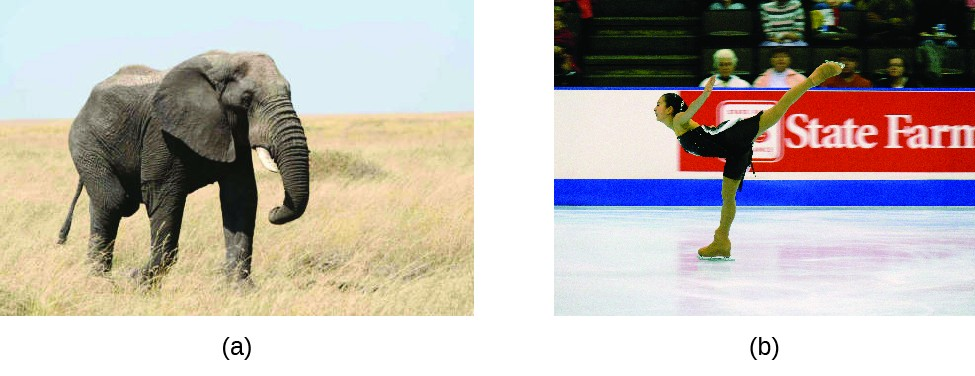Figure 3. Although (a) an elephant’s weight is large, creating a very large force on the ground, (b) the figure skater exerts a much higher pressure on the ice due to the small surface area of her skates. (credit a: modification of work by Guido da Rozze; credit b: modification of work by Ryosuke Yagi)

The SI unit of pressure is the pascal (Pa), with 1 Pa = 1 N/m2, where N is the newton, a unit of force defined as 1 kg m/s2. One pascal is a small pressure; in many cases, it is more convenient to use units of kilopascal (1 kPa = 1000 Pa) or bar (1 bar = 100,000 Pa). In the United States, pressure is often measured in pounds of force on an area of one square inch—pounds per square inch (psi)—for example, in car tires. Pressure can also be measured using the unit atmosphere (atm), which originally represented the average sea level air pressure at the approximate latitude of Paris (45°). Table 1 provides some information on these and a few other common units for pressure measurements

Table 1. Pressure Units
Unit Name and Abbreviation Definition or Relation to Other Unit
pascal (Pa) 1 Pa = 1 N/m2
recommended IUPAC unit
kilopascal (kPa) 1 kPa = 1000 Pa
pounds per square inch (psi) 1 psi = 0.060846 atm
atmosphere (atm) 1 atm = 101,325 Pa
air pressure at sea level is ~1 atm
bar (bar, or b) 1 bar = 100,000 Pa (exactly)
commonly used in meteorology
millibar (mbar, or mb) 1000 mbar = 1 bar
inches of mercury (in. Hg) 1 in. Hg = 3386 Pa =0.03342 atm
used by aviation industry, also some weather reports
torr $\text{1 torr}=\frac{\text{1}}{\text{760}}\text{atm}$
named after Evangelista Torricelli, inventor of the barometer
millimeters of mercury (mm Hg) 1 mm Hg ~1 torr

### Example 1: Conversion of Pressure Units

The United States National Weather Service reports pressure in both inches of Hg and millibars. Convert a pressure of 29.2 in. Hg into:

1. torr
2. atm
3. kPa
4. mbar

A typical barometric pressure in Kansas City is 740 torr. What is this pressure in atmospheres, in millimeters of mercury, in kilopascals, and in bar?

We can measure atmospheric pressure, the force exerted by the atmosphere on the earth’s surface, with a barometer (Figure 4). A barometer is a glass tube that is closed at one end, filled with a nonvolatile liquid such as mercury, and then inverted and immersed in a container of that liquid. The atmosphere exerts pressure on the liquid outside the tube, the column of liquid exerts pressure inside the tube, and the pressure at the liquid surface is the same inside and outside the tube. The height of the liquid in the tube is therefore proportional to the pressure exerted by the atmosphere.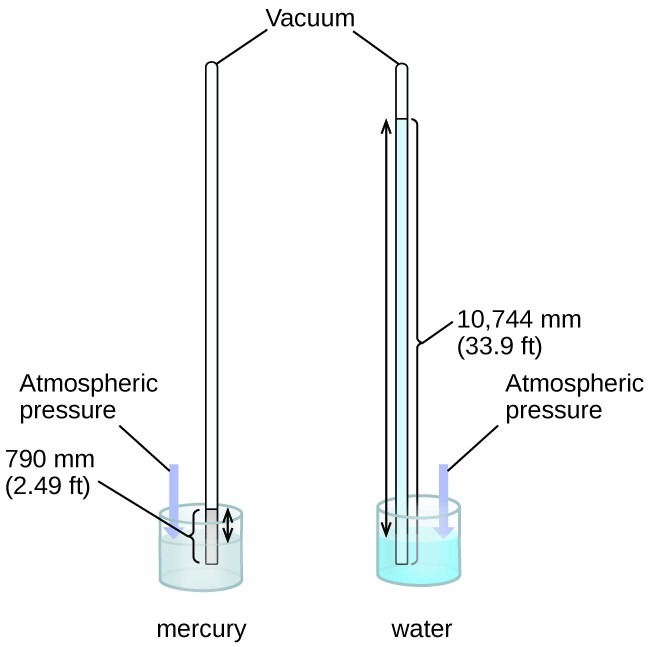Figure 4. In a barometer, the height, h, of the column of liquid is used as a measurement of the air pressure. Using very dense liquid mercury (left) permits the construction of reasonably sized barometers, whereas using water (right) would require a barometer more than 30 feet tall.

If the liquid is water, normal atmospheric pressure will support a column of water over 10 meters high, which is rather inconvenient for making (and reading) a barometer. Because mercury (Hg) is about 13.6-times denser than water, a mercury barometer only needs to be $\frac{1}{13.6}$ as tall as a water barometer—a more suitable size. Standard atmospheric pressure of 1 atm at sea level (101,325 Pa) corresponds to a column of mercury that is about 760 mm (29.92 in.) high.

## Measuring Blood Pressure

Blood pressure is measured using a device called a sphygmomanometer (Greek sphygmos = “pulse”). It consists of an inflatable cuff to restrict blood flow, a manometer to measure the pressure, and a method of determining when blood flow begins and when it becomes impeded (Figure 5). Since its invention in 1881, it has been an essential medical device. There are many types of sphygmomanometers: manual ones that require a stethoscope and are used by medical professionals; mercury ones, used when the most accuracy is required; less accurate mechanical ones; and digital ones that can be used with little training but that have limitations. When using a sphygmomanometer, the cuff is placed around the upper arm and inflated until blood flow is completely blocked, then slowly released. As the heart beats, blood forced through the arteries causes a rise in pressure. This rise in pressure at which blood flow begins is the systolic pressure—the peak pressure in the cardiac cycle. When the cuff’s pressure equals the arterial systolic pressure, blood flows past the cuff, creating audible sounds that can be heard using a stethoscope. This is followed by a decrease in pressure as the heart’s ventricles prepare for another beat. As cuff pressure continues to decrease, eventually sound is no longer heard; this is the diastolic pressure—the lowest pressure (resting phase) in the cardiac cycle. Blood pressure units from a sphygmomanometer are in terms of millimeters of mercury (mm Hg).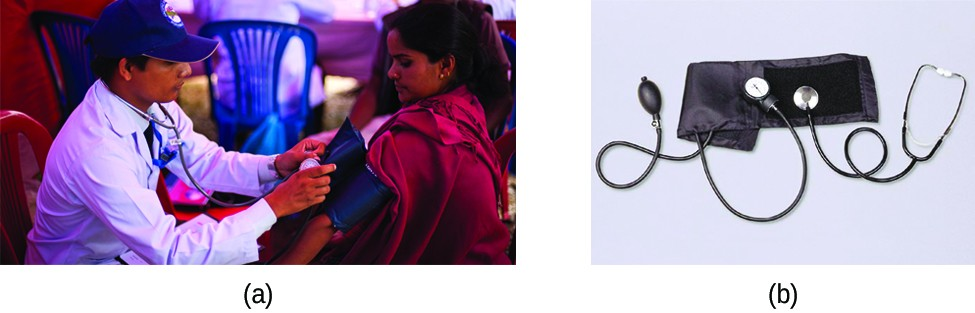Figure 5. (a) A medical technician prepares to measure a patient’s blood pressure with a sphygmomanometer. (b) A typical sphygmomanometer uses a valved rubber bulb to inflate the cuff and a diaphragm gauge to measure pressure. (credit a: modification of work by Master Sgt. Jeffrey Allen)

## Meteorology, Climatology, and Atmospheric Science

Throughout the ages, people have observed clouds, winds, and precipitation, trying to discern patterns and make predictions: when it is best to plant and harvest; whether it is safe to set out on a sea voyage; and much more. We now face complex weather and atmosphere-related challenges that will have a major impact on our civilization and the ecosystem. Several different scientific disciplines use chemical principles to help us better understand weather, the atmosphere, and climate. These are meteorology, climatology, and atmospheric science. Meteorology is the study of the atmosphere, atmospheric phenomena, and atmospheric effects on earth’s weather. Meteorologists seek to understand and predict the weather in the short term, which can save lives and benefit the economy. Weather forecasts (Figure 6) are the result of thousands of measurements of air pressure, temperature, and the like, which are compiled, modeled, and analyzed in weather centers worldwide.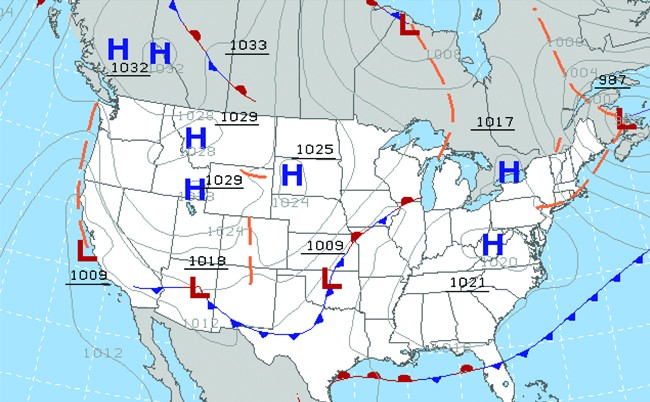Figure 6. Meteorologists use weather maps to describe and predict weather. Regions of high (H) and low (L) pressure have large effects on weather conditions. The gray lines represent locations of constant pressure known as isobars. (credit: modification of work by National Oceanic and Atmospheric Administration)

In terms of weather, low-pressure systems occur when the earth’s surface atmospheric pressure is lower than the surrounding environment: Moist air rises and condenses, producing clouds. Movement of moisture and air within various weather fronts instigates most weather events.

The atmosphere is the gaseous layer that surrounds a planet. Earth’s atmosphere, which is roughly 100–125 km thick, consists of roughly 78.1% nitrogen and 21.0% oxygen, and can be subdivided further into the regions shown in Figure 7: the exosphere (furthest from earth, > 700 km above sea level), the thermosphere (80–700 km), the mesosphere (50–80 km), the stratosphere (second lowest level of our atmosphere, 12–50 km above sea level), and the troposphere (up to 12 km above sea level, roughly 80% of the earth’s atmosphere by mass and the layer where most weather events originate). As you go higher in the troposphere, air density and temperature both decrease.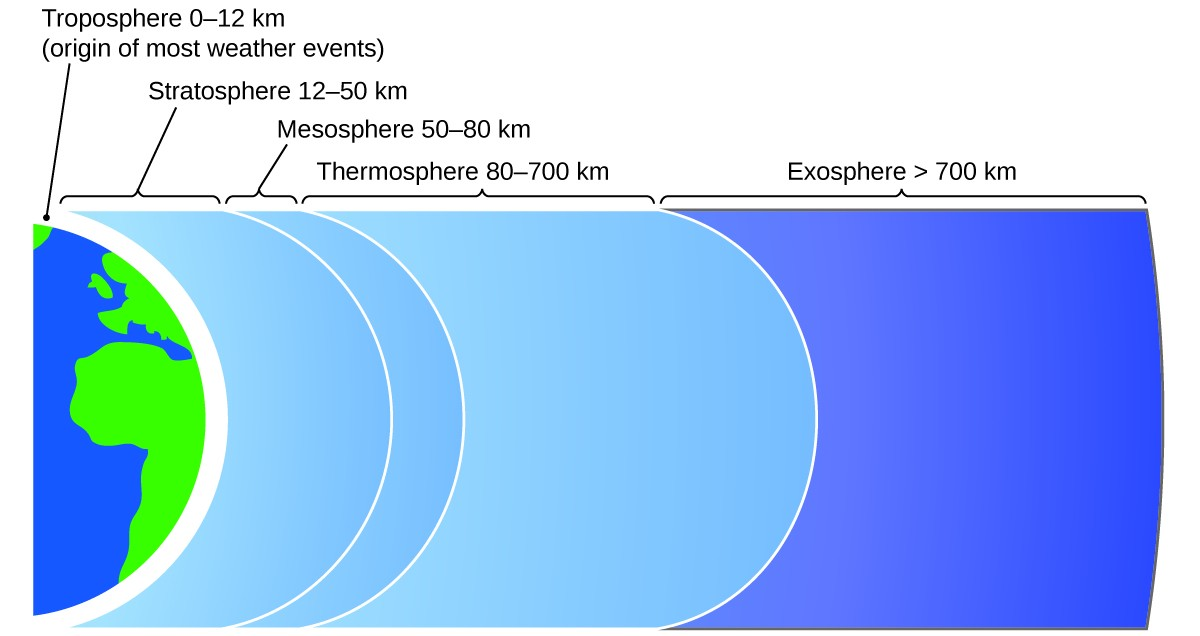Figure 7. Earth’s atmosphere has five layers: the troposphere, the stratosphere, the mesosphere, the thermosphere, and the exosphere.

Climatology is the study of the climate, averaged weather conditions over long time periods, using atmospheric data. However, climatologists study patterns and effects that occur over decades, centuries, and millennia, rather than shorter time frames of hours, days, and weeks like meteorologists. Atmospheric science is an even broader field, combining meteorology, climatology, and other scientific disciplines that study the atmosphere.

### Key Concepts and Summary

Gases exert pressure, which is force per unit area. The pressure of a gas may be expressed in the SI unit of pascal or kilopascal, as well as in many other units including torr, atmosphere, and bar. Atmospheric pressure is measured using a barometer;

### Exercises

1. Why are sharp knives more effective than dull knives (Hint: think about the definition of pressure)?
2. Why do some small bridges have weight limits that depend on how many wheels or axles the crossing vehicle has?
3. Why should you roll or belly-crawl rather than walk across a thinly-frozen pond?
4. A typical barometric pressure in Redding, California, is about 750 mm Hg. Calculate this pressure in atm and kPa.
5. A typical barometric pressure in Denver, Colorado, is 615 mm Hg. What is this pressure in atmospheres and kilopascals?
6. A typical barometric pressure in Kansas City is 740 torr. What is this pressure in atmospheres, in millimeters of mercury, and in kilopascals?
7. Canadian tire pressure gauges are marked in units of kilopascals. What reading on such a gauge corresponds to 32 psi?
8. During the Viking landings on Mars, the atmospheric pressure was determined to be on the average about 6.50 millibars (1 bar = 0.987 atm). What is that pressure in torr and kPa?
9. The pressure of the atmosphere on the surface of the planet Venus is about 88.8 atm. Compare that pressure in psi to the normal pressure on earth at sea level in psi.
10. A medical laboratory catalog describes the pressure in a cylinder of a gas as 14.82 MPa. What is the pressure of this gas in atmospheres and torr?
11. Consider this scenario and answer the following questions: On a mid-August day in the northeastern United States, the following information appeared in the local newspaper: atmospheric pressure at sea level 29.97 in., 1013.9 mbar.
1. What was the pressure in kPa?
2. The pressure near the seacoast in the northeastern United States is usually reported near 30.0 in. Hg. During a hurricane, the pressure may fall to near 28.0 in. Hg. Calculate the drop in pressure in torr.
12. Why is it necessary to use a nonvolatile liquid in a barometer or manometer?
13. How would the use of a volatile liquid affect the measurement of a gas using open-ended manometers vs. closed-end manometers?

## Glossary

atmosphere (atm): unit of pressure; 1 atm = 101,325 Pa

bar: (bar or b) unit of pressure; 1 bar = 100,000 Pa

barometer: device used to measure atmospheric pressure

pascal (Pa): SI unit of pressure; 1 Pa = 1 N/m2

pounds per square inch (psi): unit of pressure common in the US

pressure: force exerted per unit area

torr: unit of pressure; $\text{1 torr}=\frac{1}{760}\text{atm}$